Courses

# BJT As an Amplifier - 1

## 10 Questions MCQ Test Topicwise Question Bank for Electronics Engineering | BJT As an Amplifier - 1

Description
This mock test of BJT As an Amplifier - 1 for Electrical Engineering (EE) helps you for every Electrical Engineering (EE) entrance exam. This contains 10 Multiple Choice Questions for Electrical Engineering (EE) BJT As an Amplifier - 1 (mcq) to study with solutions a complete question bank. The solved questions answers in this BJT As an Amplifier - 1 quiz give you a good mix of easy questions and tough questions. Electrical Engineering (EE) students definitely take this BJT As an Amplifier - 1 exercise for a better result in the exam. You can find other BJT As an Amplifier - 1 extra questions, long questions & short questions for Electrical Engineering (EE) on EduRev as well by searching above.
QUESTION: 1

Solution:
QUESTION: 2

### The current gain of a common-emitter amplifier _____ as the load resistance is increased.

Solution:

As the load resistance is increases, collector current reduces and hence current gain (β) is decreased.

QUESTION: 3

### Cascading amplifier stages to obtain a high gain is best done with

Solution:

Since the short-circuit current gain hfe of a common-emitter stage is much greater than unity, it is possible to increase the voltage amplification by cascading such stages. Hence, in a cascade, the intermediate transistors should be connected in a common-emitter configuration.

QUESTION: 4

The h-parameter equivalent circuit is the most common representation of the transistor because

Solution:
QUESTION: 5

Assertion (A): The voltage gain of the emitter follower is very high.
Reason (R): The emitter follower increases the power level of the signal.

Solution:

The common-collector transistor configuration is called the emitter follower because its voltage gain is close to unity (low) and hence the emitter follows the input signal.
Hence, assertion is a false statement.

QUESTION: 6

For a cascade amplifier shown below, the value of voltage gain V0/Vs is given by
(Use hie = 1 km, hfe = 100, hre = 0, and hoe = 0)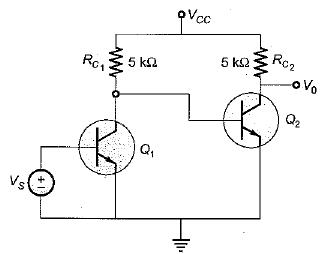Solution:

The equivalent circuit of the given cascade amplifier can be drawn as shown below.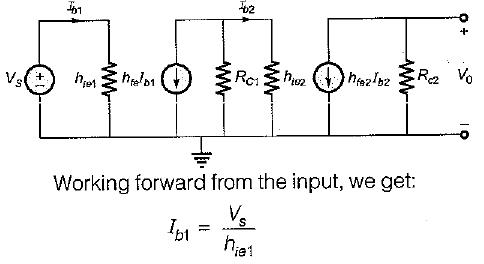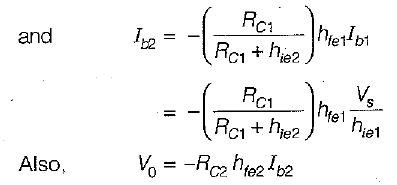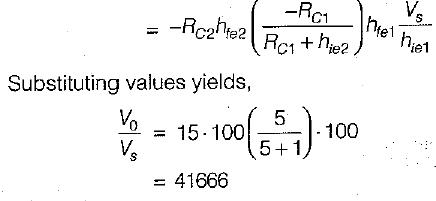QUESTION: 7

The h-parameters for the transistor shown below are given as:
hie = 10 kΩ, hfe = 100, hre = 0, and hoe = 0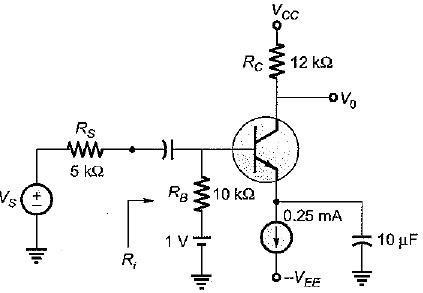The value of voltage gain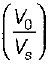and input resistance (Ri) for the circuit shown above are respectively given by

Solution:

Figure below shows the small-signal circuit (with D.C. voltage source and capacitors short circuited).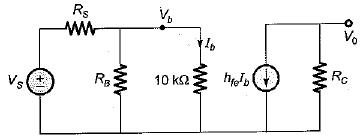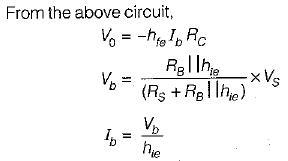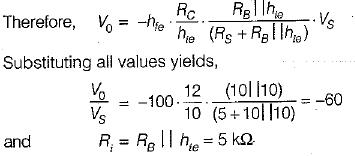QUESTION: 8

Assertion (A): The Darlington connection of three or more transistors is usually impractical.
Reason (R): The Darlington emitter follower has a higher input resistance and a voltage gain less close to unity than does a single-stage emitter follower.

Solution:

The Darlington connection of three or more transistors is usually impractical because leakage current of the first transistor is amplified by the second due to which the overall leakage current may become high.
Reason is individually a correct statement. Hence, both assertion and reason are correct but reason is not the correct explanation of assertion.

QUESTION: 9

Bootstrapping is used in emitter follower configuration to

Solution:

The input resistance of emitter follower can be increased by bootstrapping the darlington circuit through the addition of capacitor C0 between the first collector (C1) and the second emitter (E2).

QUESTION: 10

Consider the following statements associated with the use of h-parameters to describe a transistor:
1. They are easy to measure.
2. They are real numbers for all frequency range.
3. They are easily convertible from one configuration to other.
4. The smallest value of h-parameter; for a transistor configuration is that of short circuit input impedance (hi).

Which of the statements given above are correct?

Solution:

h-parameters are real numbers upto radio frequency only. The smallest value of h-parameter is that of open circuit output admittance (h0). Hence, statements 2 and 4 are not correct.